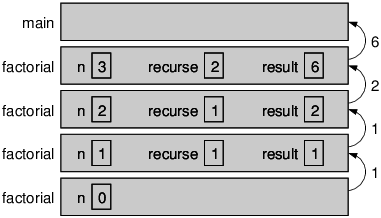# 14.7: More Recursion

Now that we have methods that return values, we have a Turing complete programming language. That means Java can compute anything computable, for any reasonable definition of “computable”. This idea was developed by Alonzo Church and Alan Turing, so it is known as the Church-Turing thesis.

To give you an idea of what you can do with the tools we have learned, let’s look at some methods for evaluating recursively-defined mathematical functions. A recursive definition is similar to a circular definition, in the sense that the definition refers to the thing being defined.

Of course, a truly circular definition is not very useful:

recursive:
An adjective used to describe a method that is recursive.

If you saw that definition in the dictionary, you might be annoyed. But if you search for recursion on Google, it displays “Did you mean: recursion” as an inside joke.

Many mathematical functions are defined recursively, because that is often the simplest way. For example, the factorial of an integer n, which is written n!, is defined like this:

\begin{align*} & 0! = 1 \\[4pt] & n! = n \cdot (n-1)! \end{align*}

Don’t confuse the mathematical symbol !, which means factorial, with the Java operator !, which means not. This definition says that factorial(0) is 1, and that factorial(n) is n * factorial(n - 1).

So factorial(3) is 3 * factorial(2); factorial(2) is 2 * factorial(1); factorial(1) is 1 * factorial(0); and factorial(0) is 1. Putting it all together, we get 3 * 2 * 1 * 1, which is 6.

If you can formulate a recursive definition of something, you can easily write a Java method to evaluate it. The first step is to decide what the parameters and return type are. Since factorial is defined for integers, the method takes an int as a parameter and returns an int. So here’s a good starting place:

public static int factorial(int n) {
return 0;
}


Next, we think about the base case. If the argument happens to be zero, we return 1.

public static int factorial(int n) {
if (n == 0) {
return 1;
}
return 0;
}

Otherwise, and this is the interesting part, we have to make a recursive call to find the factorial of n−1, and then multiply it by n.

public static int factorial(int n) {
if (n == 0) {
return 1;
}
int recurse = factorial(n - 1);
int result = n * recurse;
return result;
}


The flow of execution for this program is similar to countdown from Section 5.8. If we invoke factorial with the value 3:

Since 3 is not zero, we take the second branch and calculate the factorial of n−1...
Since 2 is not zero, we take the second branch and calculate the factorial of n−1...
Since 1 is not zero, we take the second branch and calculate the factorial of n−1...
Since 0 is zero, we take the first branch and return the value 1 immediately.
The return value (1) gets multiplied by n, which is 1, and the result is returned.
The return value (1) gets multiplied by n, which is 2, and the result is returned.
The return value (2) gets multiplied by n, which is 3, and the result, 6, is returned to whatever invoked factorial(3)..

Figure 6.7.1 shows what the stack diagram looks like for this sequence of method invocations. The return values are shown being passed back up the stack. Notice that recurse and result do not exist in the last frame, because when n == 0 the code that declares them does not execute.Figure $$\PageIndex{1}$$: Stack diagram for the factorial method.